16. The numbers p, q, r and s satisfy the following equations:

p + 2q + 3r + 4s = k 4p = 3q = 2r = s.

What is the smallest value of k for which p, q, r and s are all positive integers?

A:B:C:D:E:17. Bethany has 11 pound coins and some 20p coins and some 50p coins in her purse. The mean value of the coins is 52 pence.

Which could not be the number of coins in the purse?

A:B:C:D:E:18. P, Q and R are the three angles of a triangle, when each has been rounded to the nearest degree.

Which of the following is the complete list of possible values of P + Q + R?

A:B:C:D:E:19. How many pairs of numbers (m, n) are there such that the following statement is true?‘A regular m-sided polygon has an exterior angle of size n° and

a regular n-sided polygon has an exterior angle of size m°.’

A:B:C:D:E:20. The diagram shows a semicircle of radius 1 inside an isosceles triangle. The diameter of the semicircle lies along the ‘base’ of the triangle, and the angle of the triangle opposite the ‘base’ is equal to 2θ. Each of the two equal sides of the triangle is tangent to the semicircle.

What is the area of the triangle?

A: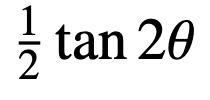B: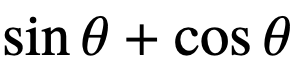C: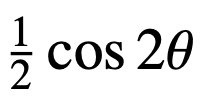D:E: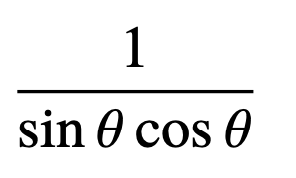21.

A: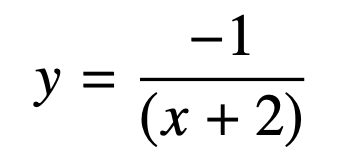B: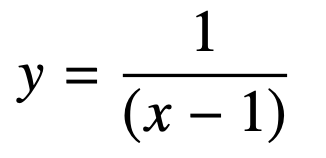C: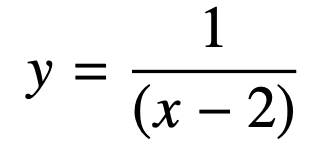D: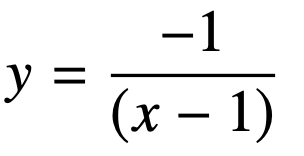E: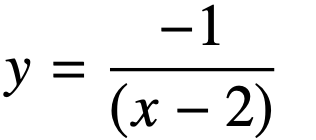22. The diagram shows two overlapping triangles; an isosceles triangle with an angle of 120° and an equilateral triangle with area 36. Two of the vertices of the equilateral triangle are midpoints of the equal sides of the isosceles triangle.

What is the total area of the shaded regions (inside the isosceles triangle but outside the equilateral triangle)?

A:B:C:D:E:23. For particular real numbers a and b, the function f is defined by f (x) = ax + b, and is such that f (f(f(x))) =27x−52.

Which of the following formulas defines the function g such that, for all values of x,g(f(x)) = x?

A: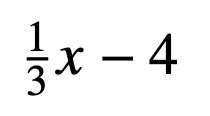B: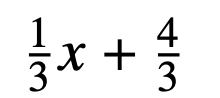C: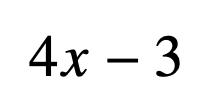D: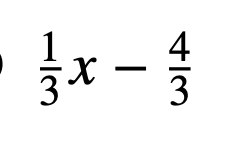E: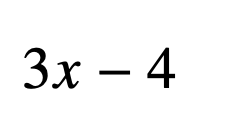A: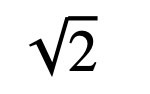B: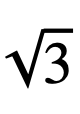C: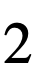D: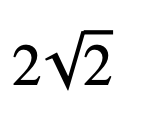E: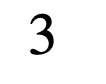25. A semicircle is inscribed in a quarter circle as shown.What fraction of the quarter circle is shaded?

A: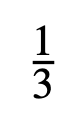B: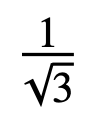C: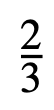D: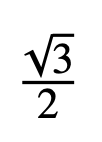E: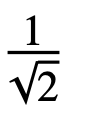Back to the top